MapThread

MapThread can be used to Apply a function to each column of a matrix as I do
in the next cell. However, before using MapThread I clear the values from all
variables.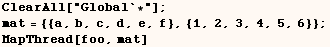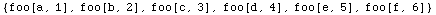MapThread is equivalent to using Map followed by Apply at level 2 (see
below).  I have to wonder why it isn't called ApplyThread.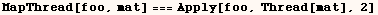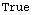In the next few lined we use MapThread to work with the tensor below.In the next line MapThread is used on the above tensor.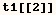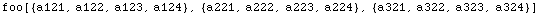MapThread can work on the tensor above with a third argument as in the next
example, and gives a very different result than the previous example.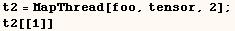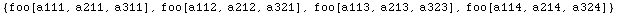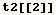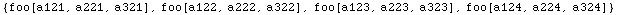An interesting application of MapThread is given below where MapThread is  used to apply a list of functions to a list of arguments.  The #& notation is  explained in the discussion of Function.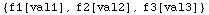Alan Hayes provided the code below to make a list of replacement rules.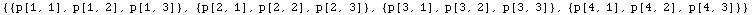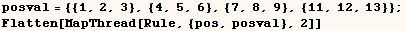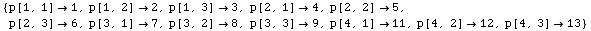Created by Mathematica  (May 16, 2004)

Back to Ted’s Tricks index page